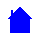## ELET1210 Course Outline

```
PLEASE NOTE:  THERE ARE TWO LECTURES PER WEEK

Course Outline:
-----------------------
Lecture 1 -  Rules of Boolean Algebra, AND - OR - NOT gates, truth tables
Lecture 2 -  Boolean identities, NAND - NOR - XOR -  Exclusive-NOR gates and simplification using the Rules
Lecture 3 -  Canonical and standard forms, Sum of Products (SOP) and
Product of Sums (POS), minterms and maxterms
Lecture 4 -  Conversion between SOP and POS form and simplification using this method
Lecture 5 -  Karnaugh mapping of 2,3,4-variable functions
Lecture 6 -  Boolean Simplification using Karnaugh mapping
Lecture 7 -  Number systems, binary and hexadecimal conversions, binary arithmetic
half adder and full adder logic diagrams and truth tables, half subtractor
Lecture 8 -  Representation of negative numbers, 1's and 2's complement, subtraction
by addition of complement, complement of functions
Lecture 9 -  Representation of Real Numbers in computers (with example of
Single Precision, Floating Point scheme used in IBM PC), coding schemes for
other purposes (Gray code, Excess-3, ASCII)
Lecture 10 -  Conclusion of Real Numbers (ranges, representation of zero and overflow,
underflow),
NAND-NAND and NOR-NOR implementations compared and contrasted.
Lecture 11 -  Encoders, Decoders, Multiplexors & Demultiplexors, Data Selectors,
truth tables
Lecture 12 -  Truth tables for multiplexors and demultiplexors, octal number system,
BCD arithmetic and a BCD adder circuit, Parity generator and checker.
Lecture 13 - 1's complement subtraction (by addition), using a multiplexor to implement
a logic function, combinational and sequential logic,
S-R latch using NOR gates and NAND gates
Lecture 14 - clocked S-R Latch using NAND gates, D type flip-flop, glitches,
J-K master-slave flip-flop
Lecture 15 - conclusion of flip-flop circuits
Lecture 16 - truth table for JK edge-triggered
flip-flop with preset and clear, Tri-State logic
Lecture 17 - State Diagram, State Table, State Equations and
flip-flop input functions, analysis of sequential circuits
Lecture 18 -  Unused states and the state diagram applied to counters, self-correction,
design of a 3-stage synchronous binary counter using a state table
and "toggle" input functions.
Lecture 19 -  3-bit parity generator and 4-bit checker, TTL (bipolar) and CMOS (fet) logic
RTL, DTL, ECL logic families, fan out, propagation delay and
power disipation

Recommended text: Digital Design by M. Morris Mano (Prentice-Hall International publication)
```ELET1210 Homepage